Change Of Base Formula: Logarithms & Proof

Lesson Transcript
Instructor: Jennifer Beddoe

Jennifer has an MS in Chemistry and a BS in Biological Sciences.

An easy way to solve logarithms with a base other than 10 is with the change of base formula. This lesson will review the basics of logarithms, and explain the change of base formula with examples, and proof of how it works. Updated: 09/06/2021

Logarithms

Let's start with a basic review of logarithms. The logarithm (log) of a number is the exponent a fixed number must be raised to in order to equal a given number. In mathematical terms: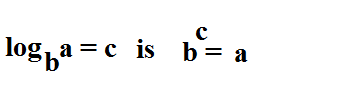Where b is the base, a is the set number, and c is the given number.

For example,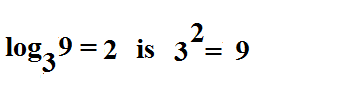Log functions are important in many areas of science, business, and engineering. For example, the Richter scale that measures the intensity of earthquakes is a logarithmic scale. The growth of bacteria is measured using log functions. And stock brokers can use log functions to predict the growth of a stock portfolio.An error occurred trying to load this video.

Try refreshing the page, or contact customer support.

Coming up next: Transformations in Math: Definition & Graph

You're on a roll. Keep up the good work!

Replay
Your next lesson will play in 10 seconds
• 0:03 Logarithms
• 0:51 The Change of Base Formula
• 3:29 Proof
• 4:31 Lesson Summary
Save Save

Want to watch this again later?

Timeline
Autoplay
Autoplay
Speed Speed

The Change of Base Formula

Often, logarithmic equations are written with a base that cannot be easily calculated. Scientific calculators are designed to calculate logs that have a base of ten. Solutions to logs with other bases can be found using charts, or simple calculations. Some are easy, like in the example above. The base is 3, and the problem is asking 'to what exponent must 3 be raised in order to equal 9?' And of course, the answer is 2. Others can be more difficult, like the following: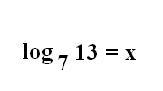When converted to exponential forms, this equation becomes 7^x = 13.This problem is impossible to do without looking through a myriad of tables or guessing a thousand (or more) times until you got close.

The easiest way to solve a problem like this is to use the change of base formula. It will allow you to convert the base of any logarithm to something more usable. Most often, you will use it to convert the base to 10, since this is what your calculator uses. The change of base formula is as follows: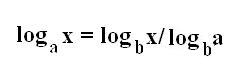To use this to solve the example problem, we can plug in the numbers to get this equation: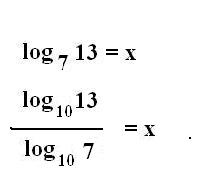Since the logs are now to the base 10, you can use your calculator to solve.

1.1134/0.845 = x
x = 1.318
Which means that 7^1.318 = 13

As with many problems in mathematics, there is a way to check your answer. Just perform the exponent calculation that results from finding the log, and if you get the correct answer, your problem is correct.

For this example, if you plug 7^1.318 into your calculator, you should get 13 - or a number very close, that easily rounds to 13.

To unlock this lesson you must be a Study.com Member.

Register to view this lesson

Are you a student or a teacher?